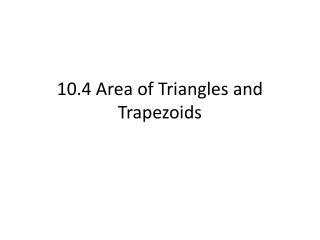DownloadDownload Presentation10.4 Area of Triangles and Trapezoids

# 10.4 Area of Triangles and Trapezoids

Télécharger la présentation## 10.4 Area of Triangles and Trapezoids

- - - - - - - - - - - - - - - - - - - - - - - - - - - E N D - - - - - - - - - - - - - - - - - - - - - - - - - - -
##### Presentation Transcript

1. 10.4 Area of Triangles and Trapezoids

2. Vocabulary Areas of Triangles and Trapezoids What You'll Learn You will learn to find the areas of triangles and trapezoids. Nothing new!

3. h b Areas of Triangles and Trapezoids Look at the rectangle below. Its area is bh square units. congruent triangles The diagonal divides the rectangle into two _________________. The area of each triangle is half the area of the rectangle, or This result is true of all triangles and is formally stated in Theorem 10-3.

4. Height Base Areas of Triangles and Trapezoids Consider the area of this rectangle A(rectangle) = bh

5. h b Areas of Triangles and Trapezoids a base of b units, and a corresponding altitude of h units, then

6. 18 mi 6 yd 23 mi Areas of Triangles and Trapezoids Find the area of each triangle: A = 207 mi2 A = 13 yd2

7. height base Because the opposite sides of a parallelogram have the same length,the area of a parallelogram is closely related to the area of a ________. Next we will look at the area of trapezoids. However, it is helpful to first understand parallelograms. rectangle height The area of a parallelogram is found by multiplying the ____ and the ______. base Base – the bottom of a geometric figure. Height – measured from top to bottom, perpendicular to the base.

8. b1 b2 h b2 b1 Areas of Triangles and Trapezoids Starting with a single trapezoid. The height is labeled h, and the bases are labeled b1 and b2 Construct a congruent trapezoid and arrange it so that a pair of congruent legs are adjacent. The new, composite figure is a parallelogram. It’s base is (b1 + b2) and it’s height is the same as the original trapezoid. The area of the parallelogram is calculated by multiplying the base X height. A(parallelogram) = h(b1 + b2) The area of the trapezoid is one-half of the parallelogram’s area.

9. b1 h b2 Areas of Triangles and Trapezoids bases of b1 and b2 units, and an altitude of h units, then

10. 20 in 18 in 38 in Areas of Triangles and Trapezoids Find the area of the trapezoid: A = 522 in2

11. Areas of Triangles and Trapezoids End of Lesson